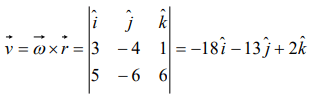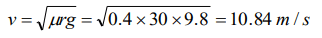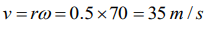## Motion in a Plane Questions and Answers Part-8

1. An aeroplane is flying with a uniform speed of 100 m/s along a circular path of radius 100 m. the angular speed of the aeroplane will be

Explanation:2. A body moves with constant angular velocity on a circle. Magnitude of angular acceleration
a) $r\omega^{2}$
b) Constant
c) Zero
d) None of the above

Explanation:3. What is the value of linear velocity, if $\vec{\omega}=3\hat{i}-4\hat{j}+\hat{k}$     and $\vec{r}=5\hat{i}-6\hat{j}+6\hat{k}$
a) $6\hat{i}+2\hat{j}-3\hat{k}$
b) $-18\hat{i}-13\hat{j}+2\hat{k}$
c) $4\hat{i}-13\hat{j}+6\hat{k}$
d) $6\hat{i}-2\hat{j}+8\hat{k}$

Explanation:4. A stone is tied to one end of a string 50 cm long is whirled in a horizontal circle with a constant speed. If the stone makes 10 revolutions in 20 s, what is the magnitude of acceleration of the stone
a) $493cm\diagup s^{2}$
b) $720cm\diagup s^{2}$
c) $860cm\diagup s^{2}$
d) $990cm\diagup s^{2}$

Explanation:5. A 100 kg car is moving with a maximum velocity of 9 m/s across a circular track of radius 30 m. The maximum force of friction between the road and the car is
a) 1000 N
b) 706 N
c) 270 N
d) 200 N

Explanation:6. The maximum speed of a car on a road–turn of radius 30 m, if the coefficient of friction between the tyres and the road is 0.4, will be
a) 10.84 m/sec
b) 9.84 m/sec
c) 8.84 m/sec
d) 6.84 m/sec

Explanation:7. The angular velocity of a wheel is 70 rad/sec. If the radius of the wheel is 0.5 m, then linear velocity of the wheel is
a) 70 m/s
b) 35 m/s
c) 30 m/s
d) 20 m/s

Explanation:8. A cyclist goes round a circular path of circumference 34.3 m in $\sqrt{22}$ sec. the angle made by him, with the vertical, will be
a) $45^{\circ}$
b) $40^{\circ}$
c) $42^{\circ}$
d) $48^{\circ}$

Explanation:9. A particle of mass M is moving in a horizontal circle of radius R with uniform speed V. When it moves from one point to a diametrically opposite point, its
a) Kinetic energy changes by $MV^{2}\diagup 4$
b) Momentum does not change
c) Momentum changes by 2MV
d) Kinetic energy changes by $MV^{2}$